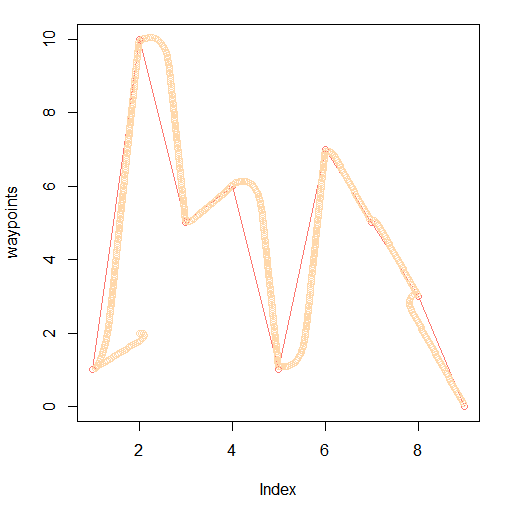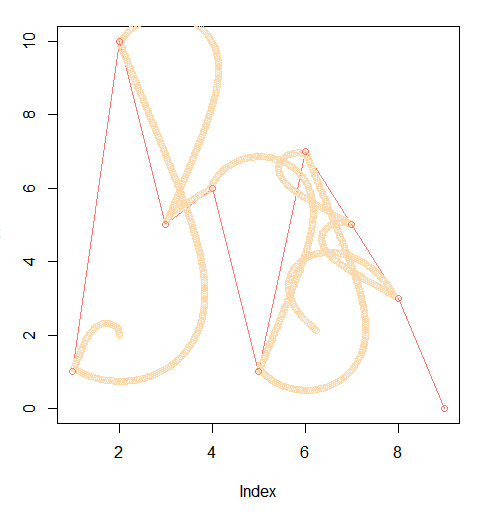Simulating a Line-Following Robot in R

I’ve been reading up on controlling mobile robots, and built a simple robotic movement simulator in R, using R graphing libraries. The motivation for doing this is to practice setting up the math for controlling a robot, without having to build a physical device. Starting with an over-simple model allows learning a bit at a time, building up to a full solution. The model sets up a series of waypoints (red), where the robot’s path is drawn over-top.The robot’s position is modeled as a combination of point and direction, and controlled using requested velocity and angle. The code below simply accepts the command and applies it exactly, although a more realistic simulation would run this through a probability function, to simulate slipping or sliding.

```move <- function(pos, command) {
loc <- c(pos\$point, pos\$point) +
c(command\$speed * cos(command\$angle),
command\$speed * sin(command\$angle));
list(point = c(loc, loc),
angle = command\$angle);
}
```

Next, we defined a few helper functions work on angles. Find the angle of a point over the horizon:

```angle <- function(goal, p) {
atan( (goal - p) / (goal - p ) )
}
```

A second function to ensure angles are between -π and π:

```angle2 <- function(theta) {
atan2(sin(theta), cos(theta));
}
```

And a third, to find the distance between two points:

```distance <- function(a, b) {
sqrt(sum((a - b) * (a - b)))
}
```

The real work is done by a simulation function, which accepts a starting position and angle, waypoint locations, a controller function, and the number of iterations to test. This graphs the waypoints and each robot position. As implemented, it doesn't render anything until the end of the simulation.

This function tests how far the robot is from the waypoint. If it gets close, it sets the next waypoint as a goal. At each iteration, the simulation provides the robot position and previous command information to a controller, which then uses that information as it wishes to decide how to move. Retaining the previous command allows the controller to track error over time, and re-adjust as necessary.

This contrived example doesn't simulate sensor inaccuracies, and thus a controller can assume it knows the exact locations of the waypoints relative to the current position. In a realistic scenario, a robot would need survey sensor data to continually refine location data.

```simulate <- function(waypoints, start, angle, f, iter) {
plot(waypoints, type="o", col="#FD7871");
command <- list(speed = 0,
angle = 0);
waypoint_idx <- 1;

position <- list(point = start,
angle = angle);

for (i in 1:iter)
{
goal <- c(waypoint_idx, waypoints[waypoint_idx]);

command <- f(position, goal, command);

points(x = position\$point, y = position\$point,
type = "o", col = "#FFD8AB");

position <- move(position, command);

if (distance(position\$point, goal) < 0.1) {
waypoint_idx <- waypoint_idx + 1;
command <- list(speed = 0, angle = 0, err = 0);
}
}

for(i in 1:length(waypoints)) {
points(x = i, y = waypoints[i], type = "o", col = "#FD7871")
}
}
```

This is an example controller, which works entirely by modifying the current angle. This controller is an attempt to implement a PID-controller, which uses the current error, net error, and change in error over time to adjust the output angles. This could modify velocity over time, although in this case it uses a constant velocity. A realistic simulation scenario might accept the output of this controller, and limit angular and velocity changes. Depending on the actual robotics hardware, the angular changes might be discretized. Velocity increases might be limited to acceleration, rather than deceleration, like cruise control in a car.

```controller <- function(position, goal, last) {
v <- -.05;
err <- angle(position\$point, goal) - position\$angle;
neterr <- last\$err + err;

list(speed = v,
angle = 5 * angle2(position\$angle + err),
err = neterr);
}
```

Tuning constant multipliers in the controller allows a variety of over and under-correction strategies, shown below. The examples I produced are not textbook examples, which suggests a defect in the simulation technique, but still represents and entertaining look at the way these things can fail. In the first example, the robot clearly under-corrects:In the second it makes odd angle choices. Other possible defects are weaving - an overall accurate path, but inability to recognize how close it is to correctness. In this situation, error over time can be tracked to prevent this behavior.The source is available on Github.

3 Replies to “Simulating a Line-Following Robot in R”

1.Weng Fu says:

Please post a Visual Basic 6 source code version for this project please.

2.anonymous says:

@Weng Fu: huh, vb6?

1.admin says:

I assumed that was a joke…